# Practice Counting Of Figures Questions and Answers

## Counting of Figures

Counting of figures forms an important part of Non-verbal reasoning. This chapter forms a part of the syllabus of various government and bank exams- SSC CGL, IBPS SO, IBPS PO, SBI PO, RBI Assistant, RBI Grade B and many others.

This topic is designed to test the analytical ability of the candidates. The figures which are asked for counting can be a straight line, triangle, square, rectangle,polygon etc. To find the accurate answer  for such questions, firstly, a candidate needs to find the required figures formed by an individual section of the given figure, then the figure formed by the combination of two figures and so on.

### What is meant by Counting of Figures?

Counting of figures can be explained as the realisation of simple geometrical plane figures from a complex figure.

### Types of Questions on Counting of Figures

#### Counting of Straight Lines

In such types of questions, a candidate is required to find out the number of straight lines in the given figure.

Straight Line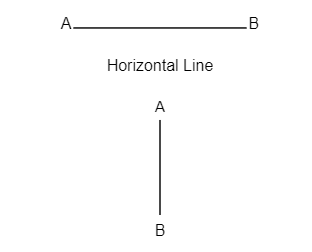A straight line is a set of points that extends endlessly in both directions.

AB

Horizontal Line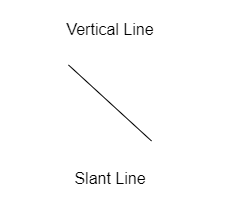A

B

Vertical Line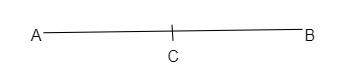Slant Line

Counting Method of Straight Line

Let’s consider the given line (AB).

AB

C

On counting , it will be counted as one line, i.e AB and not as two straight lines AC and CB.

Let’s understand with an example-

Q1) How many straight lines are there in the figure given below?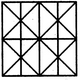Solution: 14

Explanation: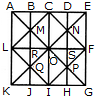The vertical lines are AK, BJ, CI, DH and EG i.e. 5 in number.

The horizontal lines are AE, LF and KG i.e. 3 in number.

The slanting lines are LC, CF, FI, LI, EK and AG i.e. 6 in number.

Thus, there are 5 + 3 + 6 = 14 straight lines in the figure.

#### Counting of Triangles

In these types of questions, a candidate is required to find the number of triangles in the given question.

A triangle is a closed figure bounded by three sides. ABC is a triangle.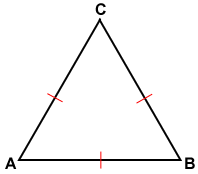Counting method of Triangles

• Smallest triangles are counted first.
• At the second step those triangles are counted which are formed with the two triangles and further counting goes on in the same way i.e, triangles formed with three, four,... triangles are counted one after another.
• Largest triangle is counted at the final step.

Note- Counting can also be done in the reverse order i.e., counting in order of largest to smallest.

Let’s understand with an example-

Q1) How many triangles are there in the following figure?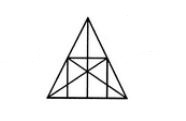Solution: 36

Explanation: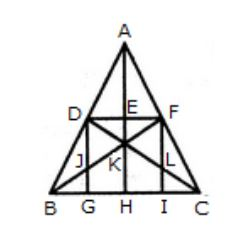There are some triangles that are easily identifiable. They are triangles (A D E), (A E F), (D E K), (E F K), (D J K), (F L K), (D J B), (F L C), (B J G), and (L I C) as you can see in the figure. The number of these triangles is ten.

There are other triangles composed of two components. They are triangles (A D F), (A F K), (D F K), (A D K), (D K B), (F C K), (B K H), (K H C), (D G B), and (F I C).

Similarly, we can find the triangles that are made up of three components. They are the triangles (D F J), and (D F L).

Also, the triangles that have four components are (A B K), (A C K), (B F I), (C D G), (D F B), (D F C) and (B K C). Thus they are seven in number. There are no triangles that have five components.

Now we can also find the triangles that are composed of six components. They are the triangles (A B H), (A C H), (A B F), (A C D), (B F C), and (C D B). Thus they are six in number.

At last, we see that there are no triangles with seven, eight, nine, ten or eleven components. And there is only one triangle which has all the twelve components i.e. the triangle A B C. Therefore, the total number of triangles in the above figure is 10 + 10 + 2 + 7 + 6 + 1 = 36.

A quadrilateral is a closed figure formed by joining four straight lines.

The different types of quadrilaterals are discussed below-

#### Parallelogram

A parallelogram has-

• Four sides
• Two unequal diagonal i.e, AC and BD
• Opposite sides are equal i.e, AB=DC and AD=BC
• Opposite angles are equal ∠ADB=∠ABC and ∠DAB=∠BCD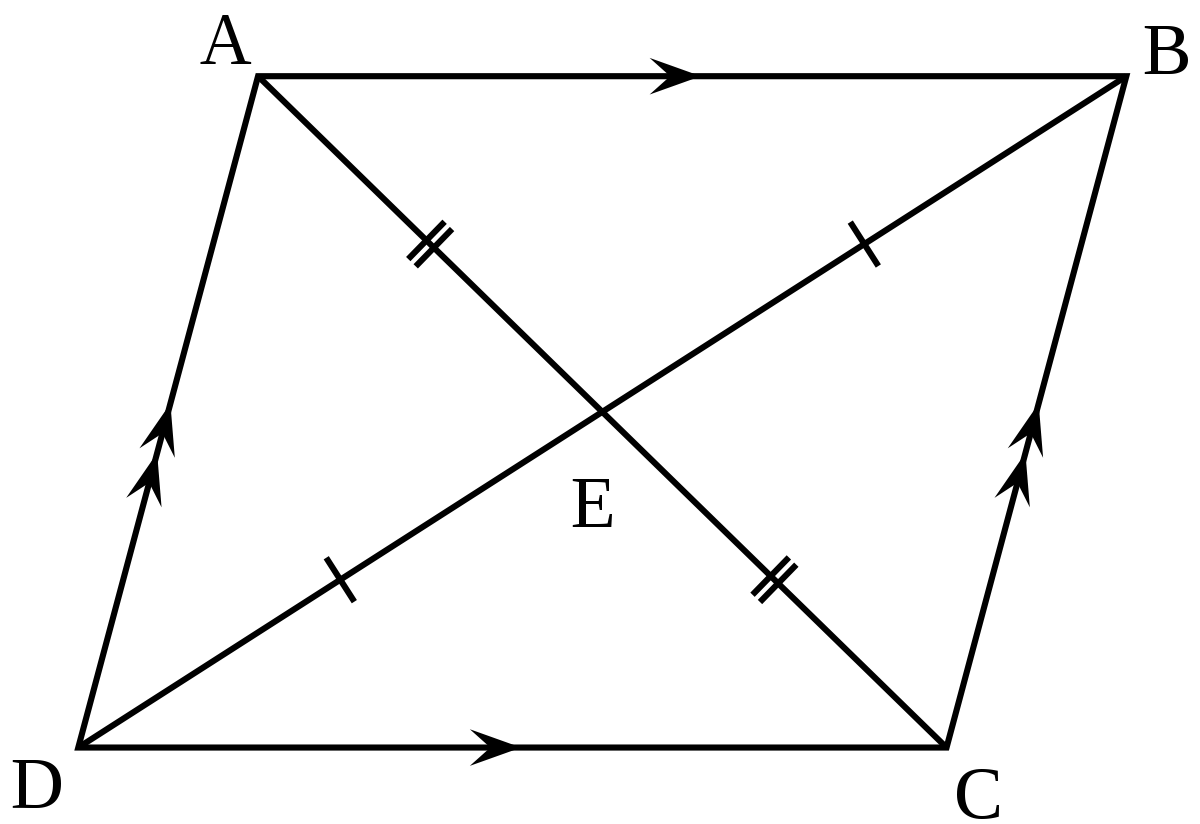Counting Method of Parallelogram

• Smallest parallelograms are counted first.
• At the second step those parallelograms are counted which are bigger than the smallest ones. Further, steps go on in the same way.

Note- Counting can also be done by supposing different bases given in the figure.

Let’s look at an example-

Q1) How many parallelograms are there in the given figure?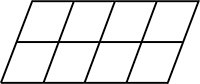Solution: 30

Explanation:

First, we will label the different points in the figure as shown. Parallelograms in the outer figure are ABLK, BCML, CDNM, DEON, ACMK, CEOM, ADNK, BEOL and AEOK.

In the top horizontal strip, there are 10 parallelograms. They are- ABGF, BCHG, CDIH, DEJI, ACHF, BDIG, CEJH, ADIF, BEJG and AEJF. Similarly, in the bottom horizontal strip, the following 10 parallelograms are there- FGLK, GHML, HINM, IJON, GINL,HJOM, FINM, GJOL and FJOK.

So, the total number of parallelograms= 10 + 10 + 10 = 30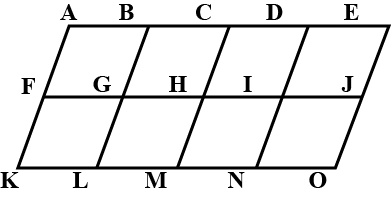#### Rectangle

Rectangle has-

• Four sides
• Opposite sides are equal i.e, AB=CD and AD=BC.
• Equal diagonals i.e, AC=BD
• Each of the four angles are equal to 90°.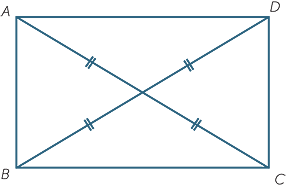Counting Method of Rectangle

• Smallest rectangles are counted first.
• At the second step those rectangles are counted which are formed with two rectangles and further counting goes on in the same way i.e, rectangles formed with three, four,… rectangles are counted one after the other.

Let’s understand with the help of an example-

Q1) How many rectangles are there in the figure given below?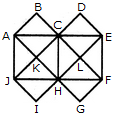Solution: 20

Explanation:

The rectangles composed of two components each are HIJE, EKJ,F, FMNG, GPQH, AEOH, EBFO, OFCG and HOGD i.e. 8 in number.

The rectangles composed of four components each are ABFH, BCGE, CDHF, DAEG and EFGH i.e. 5 in number.

The rectangles composed of six components each are IJFG, KLGH, MNHE and PQEF i.e. 4 in number.

The rectangles composed of eight components each are IJMN, KLPQ and ABCD i.e. 3 in number.

Thus, there are 8 + 5 + 4 + 3 = 20 rectangles in the given figure.

(Here, the squares are also counted amongst rectangles)#### Squares

A square has-

• Four equal sides i.e, AD=DC=CB=BA
• Equal diagonals i.e, AC=BD
• Each of the four angles are equal to 90°.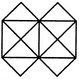Counting Method of Squares

• Smallest squares are counted first.
• At the second step those squares are counted which are formed with two squares and further counting goes on in the same way i.e, rectangles formed with three, four,… squares are counted one after the other.

Let’s look at an example-

Q1) Count the number of squares in the given figure.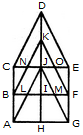Solution: 27

Explanation:

The simplest squares are EFRQ, MQYX, QRZY, RNSZ, LXWK, XYA1W, YZB1A1, ZSTB1, SGHT, WA1VP, A1B1UV, B1TOU and VUIJ i.e. 13 in number.

The squares having two components each are AEYL, FBGZ, KA1JD and B1HCI i.e. 4 in number.

The squares having four components each are MRB1W, QNTA1 XZUP and YSOV i.e. 4 in number.

The squares having seven components each are AFB1K, EBHA1 LZID and YGCJ i.e. 4 in number.

There is only one square i.e. MNOP is composed of nine components.

There is only one square i.e. ABCD is composed of seventeen components.

There are 13+ 4 + 4 + 4+1 + 1 = 27 squares in the figure.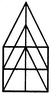#### Counting of Polygons

Polygons have more than four sides.

Counting Method of Polygons

• Smallest polygons are counted first.
• After counting smaller polygons, other polygons are counted according to increasing order of their size.

Let’s understand with the help of an example-

Q1) Count the number of convex pentagons in the given figure below.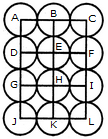Solution: 12

Explanation:

A convex pentagon has no angles pointing inwards. More precisely, no internal angles can be more than 180°.

The figure may be labeled as shown.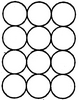The pentagons in the figure are ABDFH, CDFHB, EFHBD, GHBDF, ACDFG, CEFHA, EGHBC, GABDE, BDEGH, DFGAB, FHACD and HBCEF. Clearly, these are 12 in number.

#### Counting of Circles

In such questions, the candidates are required to find the number of circles in the given figure.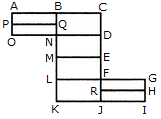Counting Method of Circle

• Keep writing numbers one by one inside the circles starting from 1 i.e, for the 1st circle put 1, for 2nd circle put 2 and so on.
• The number that is put for the last circle is the required number of circles.

Let’s look at an example-

Q1) Find the number of circles given in the figure below.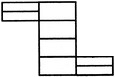Solution: 13

Explanation:

There are 13 circles in the given figure. This is clear from the adjoining figure in which the centers of all the circles in the given figure have been numbered from 1 to 13.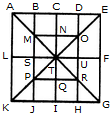### Sample Questions on Counting of Figures

Q1) count the number of squares in the figure below.• 13
• 16
• 19
• 20

Solution: b

Explanation:

The figure may be labelled as shown.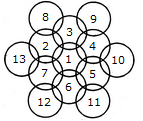The simplest squares are BCNM, CDON, PQIJ and QRHI i.e. 4 in number.

The squares composed of two components each are MNTS, NOUT, STQP and TURQ i.e. 4 in number.

The squares composed of five components each are ACTL, CEFT, TFGI and LTIK i.e. 4 in number.

The squares composed of six components each are BDUS and SUHJ i.e. 2 in number.

There is only one square i.e. MORP is composed of eight components.

There is only one square i.e. AEGK is composed of twenty components.

Total number of squares in the figure = 4 + 4 + 4 + 2+1 + 1 = 16.

Q2) How many rectangles are there in the figure below?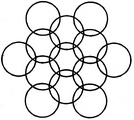• 8
• 17
• 18
• 20

Solution: c

Explanation:

The figure may be labelled as shown.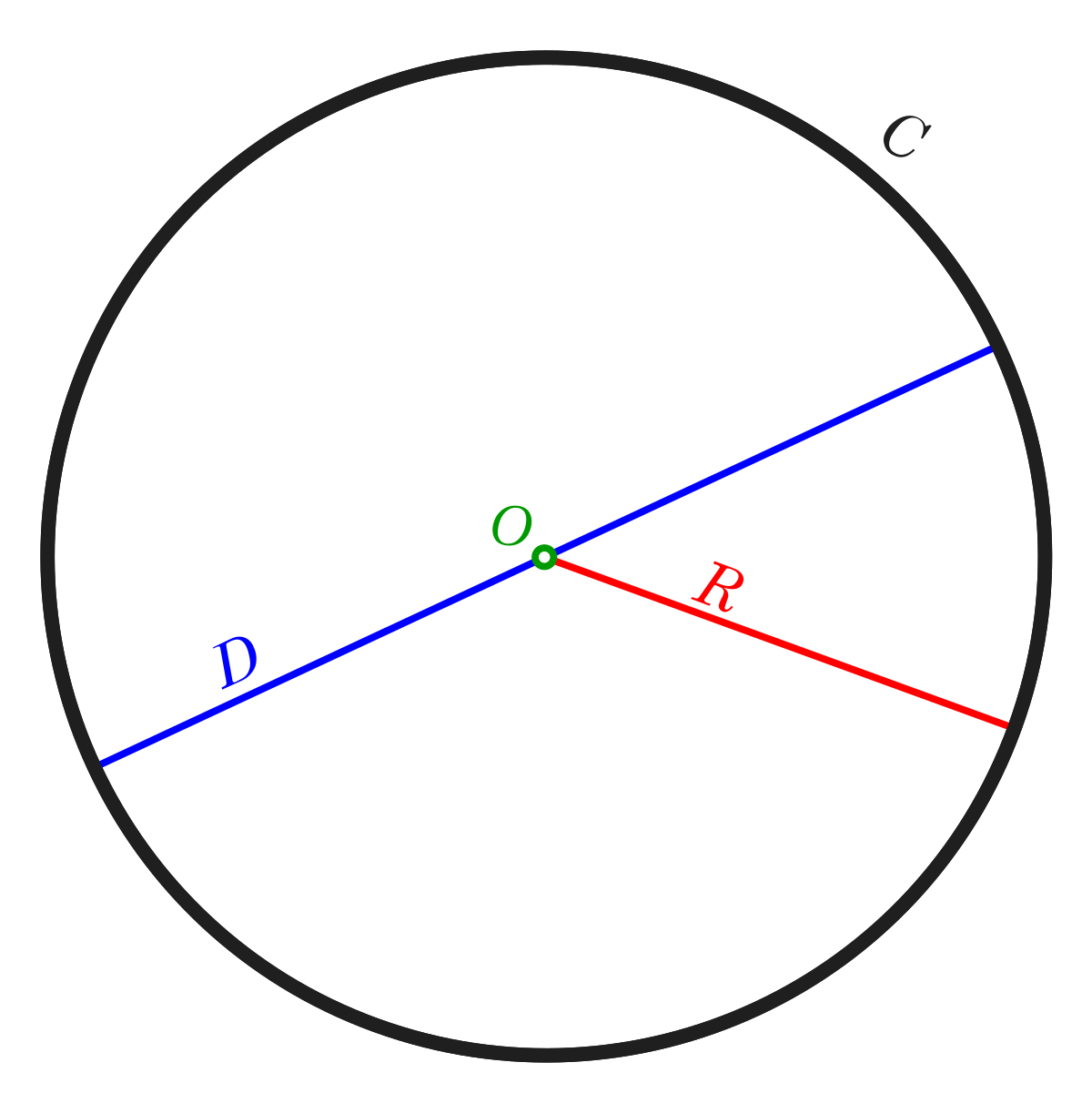The simplest rectangles are ABQP, PQNO, BCDN, NDEM, MEFL, LFJK, FGHR and RHIJ i.e. 8 in number.

The rectangles composed of two components each are ABNO, BCEM, NDFL, MEJK and FGIJ i.e. 5 in number.

The rectangles composed of three components each are ACDO, BCFL, NDJK and LGIK i.e. 4 in number.

There is only one rectangle i.e. BCJK is composed of four components.

Total number of rectangles in the figure = 8 + 5 + 4 + 1 = 18.

Q3) In the figure given below, if the centers of all the circles are joined by horizontal and vertical lines, then find the number of squares that can be formed.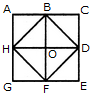• 6
• 7
• 8
• 1

Solution: c

Explanation:

The figure may be labelled as shown.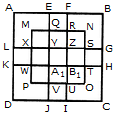We shall join the centres of all the circles by horizontal and vertical lines and then label the resulting figure as shown.

The simplest squares are ABED, BCFE, DEHG, EFIH, GHKJ and HILK i.e. 6 in number.

The squares composed of four simple squares are ACIG and DFLJ i.e. 2 in number.

Thus, 6 + 2 = 8 squares will be formed.

Q4) How many triangles & parallelograms are there in the following figure?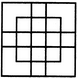• 21, 17
• 19, 13
• 21, 15
• 19, 17

Solution: a

Explanation: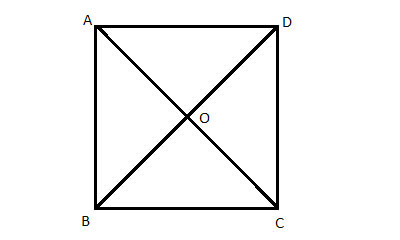Triangles:

The simplest triangles are KJN, KJO, CNB, OEF, JIL, JIM, BLA and MFG i.e. 8 in number.

The triangles composed of two components each are CDJ, EDJ, NKO, JLM, JAH and JGH i.e. 6 in number.

The triangles composed of three components each are BKI, FKI, CJA and EJG i.e. 4 in number.

The triangles composed of four components each are CDE and AJG i.e. 2 in number.

The only triangle composed of six components is BKF.

Thus, there are 8 + 6 + 4 + 2 + 1 = 21 triangles in the given figure.

Parallelograms :

The simplest parallelograms are NJLB and JOFM i.e. 2 in number.

The parallelograms composed of two components each are CDKB, DEFK, BIHA and IFGH i.e.4 in number.

The parallelograms composed of three components each are BKJA, KFGJ, CJIB and JEFI i.e.4 in number.

There is only one parallelogram i.e. BFGA is composed of four components.

The parallelograms composed of five components each are CDJA, DEGJ, CJHA and JEGH i.e.4 in number.

The only parallelogram composed of six components is CEFB.

The only parallelogram composed of ten components is CEGA.

Thus, there are 2 + 4 + 4 + 1 + 4+ 1 + 1 = 17 parallelograms in the given figure.

(Here, the squares and rectangles are also counted amongst the parallelograms).

Q5) What is the minimum number of straight lines required to construct the figure given below?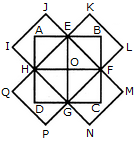• 11
• 13
• 15
• 21

Solution: b

Explanation: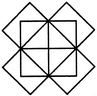The horizontal lines are AE and JF i.e. 2 in number. The vertical lines are AJ, CH and EF i.e. 3 in number.

The slanting lines are AG, BF, JD, IE, AB, DE, JI and FG i.e. 8 in number.

Total number of straight lines needed to construct the figure = 2 + 3 + 8 = 13.

### Conclusion

Counting of figures is a tricky topic as it involves many concepts and calculations. However, candidates can ace this topic by practicing a different variety of questions. Candidates can expect 2 to 3 questions in competitive exams.

×
×